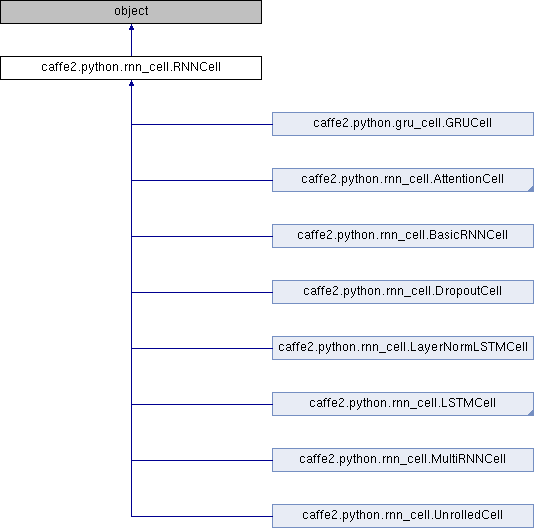Caffe2 - Python API A deep learning, cross platform ML framework
caffe2.python.rnn_cell.RNNCell Class Reference
Inheritance diagram for caffe2.python.rnn_cell.RNNCell:## Public Member Functions

def __init__ (self, name=None, forward_only=False, initializer=None)

def initializer (self)

def initializer (self, value)

def scope (self, name)

def apply_over_sequence (self, model, inputs, seq_lengths=None, initial_states=None, outputs_with_grads=None)

def apply (self, model, input_t, seq_lengths, states, timestep)

def apply_override (self, model, input_t, seq_lengths, timestep, extra_inputs=None)

def prepare_input (self, model, input_blob)

def get_output_state_index (self)

def get_state_names (self)

def get_state_names_override (self)

def get_output_dim (self)

name

recompute_blobs

forward_only

## Detailed Description

```Base class for writing recurrent / stateful operations.

One needs to implement 2 methods: apply_override
and get_state_names_override.

As a result base class will provice apply_over_sequence method, which
allows you to apply recurrent operations over a sequence of any length.

As optional you could add input and output preparation steps by overriding
corresponding methods.
```

Definition at line 48 of file rnn_cell.py.

## Member Function Documentation

 def caffe2.python.rnn_cell.RNNCell.apply_override ( self, model, input_t, seq_lengths, timestep, extra_inputs = `None` )
```A single step of a recurrent network to be implemented by each custom
RNNCell.

model: ModelHelper object new operators would be added to

input_t: singlse input with shape (1, batch_size, input_dim)

seq_lengths: blob containing sequence lengths which would be passed to
LSTMUnit operator

states: previous recurrent states

timestep: current recurrent iteration. Could be used together with
seq_lengths in order to determine, if some shorter sequences
in the batch have already ended.

extra_inputs: list of tuples (input, dim). specifies additional input
which is not subject to prepare_input(). (useful when a cell is a
component of a larger recurrent structure, e.g., attention)
```

Definition at line 192 of file rnn_cell.py.

 def caffe2.python.rnn_cell.RNNCell.get_output_dim ( self )
```Specifies the dimension (number of units) of stepwise output.
```

Definition at line 251 of file rnn_cell.py.

 def caffe2.python.rnn_cell.RNNCell.get_output_state_index ( self )
```Return index into state list of the "primary" step-wise output.
```

Definition at line 229 of file rnn_cell.py.

 def caffe2.python.rnn_cell.RNNCell.get_state_names ( self )
```Returns recurrent state names with self.name scoping applied
```

Definition at line 235 of file rnn_cell.py.

 def caffe2.python.rnn_cell.RNNCell.get_state_names_override ( self )
```Override this function in your custom cell.
It should return the names of the recurrent states.

It's required by apply_over_sequence method in order to allocate
recurrent states for all steps with meaningful names.
```

Definition at line 241 of file rnn_cell.py.

 def caffe2.python.rnn_cell.RNNCell.prepare_input ( self, model, input_blob )
```If some operations in _apply method depend only on the input,
not on recurrent states, they could be computed in advance.

model: ModelHelper object new operators would be added to

input_blob: either the whole input sequence with shape
(sequence_length, batch_size, input_dim) or a single input with shape
(1, batch_size, input_dim).
```

Definition at line 216 of file rnn_cell.py.

The documentation for this class was generated from the following file: